### CLEAN Function – Clean Text – Excel, VBA & Google Sheets

This tutorial demonstrates how to use the Excel CLEAN Function in Excel to remove all non-printable characters.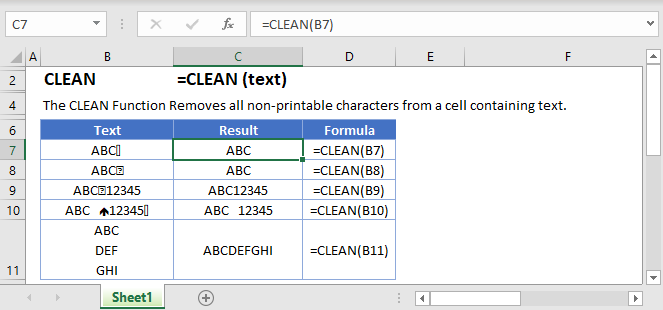## CLEAN Function Overview

The CLEAN Function Removes all non-printable characters from a cell containing text.

To use the CLEAN Excel Worksheet Function, select a cell and type: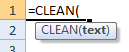(Notice how the formula inputs appear)

### CLEAN function Syntax and inputs:

`=CLEAN(text)`

text – A string of text.

AutoMacro - VBA Code Generator

## How to use the CLEAN Function in Excel:

The CLEAN function removes all non-printable from ASCII (American Standard Code for Information Interchange) character 0 to 31. One example of it is removing line breaks.

`=CLEAN(B3)`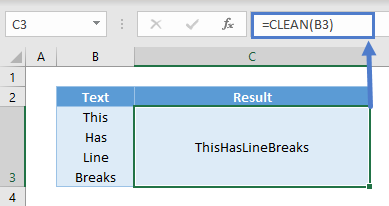## Other Non-Printable Characters

All of our characters in the keyboard has a specific ASCII (American Standard Code for Information Interchange) code to it. You could use CODE to find out what is the ASCII code of the character. Some of the unprintable ones from 1 to 31 are these below you can remove with CLEAN: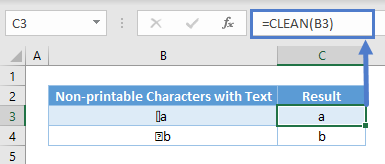If you test out the ASCII code numbers, you will see that many of them look identical. They are not meant to be used in Excel but for other programs.

## CLEAN with Number/Dates

Do note that CLEAN is a text function. Upon using CLEAN, the result is a text. For instance, you won’t be able to sum up these numbers in cell E3 after using CLEAN.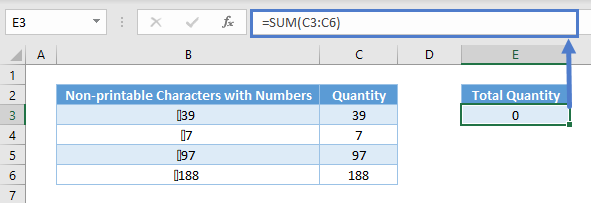The same thing happens for dates as they are recognized as serial numbers and not text. You may not need to sum dates, but it doesn’t work well in filters and PivotTables.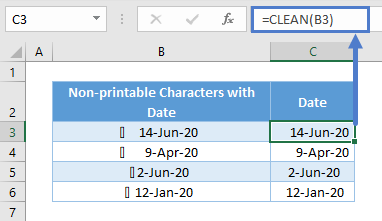To overcome the issues above, you can use VALUE to convert from text to values.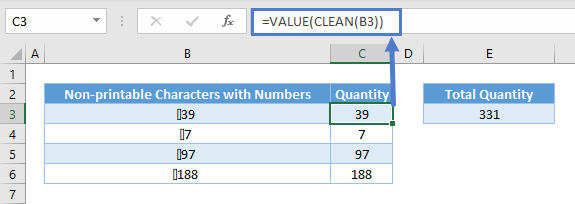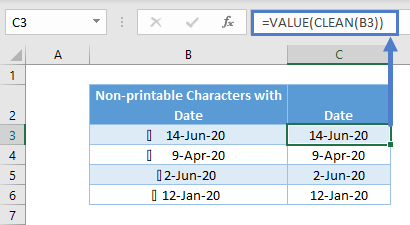## CLEAN VS TRIM

CLEAN function applicable only for non-printable characters, So if you need to remove space and other characters we need to use TRIM. refer below table to get idea about different between TRIM and CLEAN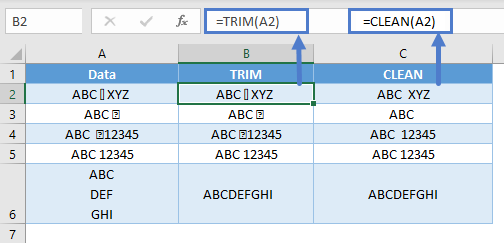## CLEAN in Google Sheets

The CLEAN Function works exactly the same in Google Sheets as in Excel: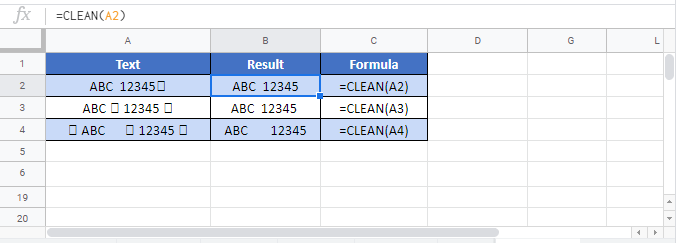## CLEAN Examples in VBA

You can also use the CLEAN function in VBA. Type:`application.worksheetfunction.clean(text)`

Executing the following VBA statements

```Range("B2") = Application.WorksheetFunction.Clean(Range("A2"))
Range("B3") = Application.WorksheetFunction.Clean(Range("A3"))
Range("B4") = Application.WorksheetFunction.Clean(Range("A4"))
Range("B5") = Application.WorksheetFunction.Clean(Range("A5"))
Range("B6") = Application.WorksheetFunction.Clean(Range("A6"))
Range("B7") = Application.WorksheetFunction.Clean(Range("A7"))
Range("B8") = Application.WorksheetFunction.Clean(Range("A8"))
Range("B9") = Application.WorksheetFunction.Clean(Range("A9"))
Range("B10") = Application.WorksheetFunction.Clean(Range("A10"))
Range("B11") = Application.WorksheetFunction.Clean(Range("A11"))
Range("B12") = Application.WorksheetFunction.Clean(Range("A12"))
Range("B13") = Application.WorksheetFunction.Clean(Range("A13"))
Range("B14") = Application.WorksheetFunction.Clean(Range("A14"))
Range("B15") = Application.WorksheetFunction.Clean(Range("A15"))
Range("B16") = Application.WorksheetFunction.Clean(Range("A16"))
Range("B17") = Application.WorksheetFunction.Clean(Range("A17"))
Range("B18") = Application.WorksheetFunction.Clean(Range("A18"))
Range("B19") = Application.WorksheetFunction.Clean(Range("A19"))
Range("B20") = Application.WorksheetFunction.Clean(Range("A20"))
Range("B21") = Application.WorksheetFunction.Clean(Range("A21"))```

will produce the following results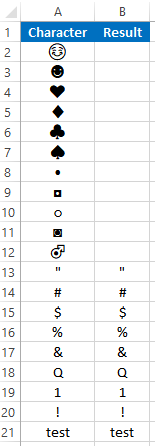For more information about the CLEAN Formula visit the Microsoft Website.

## Excel Practice Worksheet

Practice Excel functions and formulas with our 100% free practice worksheets!

• Automatically Graded Exercises
• Learn Excel, Inside Excel!